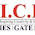## Sunday, April 28, 2013

### a. (3^(h + 1) - 1 )/ 3          b. (3^h - 1) / 2         c. (3^h - 1 ) /  3           d. (3^(h + 1) - 1)/ 2

The answer is d. (3^(h + 1) - 1) /2

Ternary tree is like a binary tree where each node can have a maximum of 3 children.

At height 0 there is one node, at height 1 there are 3 nodes, at height 2 there are 3^2 nodes and the sequence goes on,

1, 3, 3^2, 3^3,.........................................3^h

if we solve this geometric progression, the answer will be (3^(h + 1) - 1) / (3 - 1)  = ( 3^(h + 1) - 1) / 2

The maximum number of nodes in an 'm' ary tree with height h, where each node in the tree can have a maximum of m children is (m^(h+1) - 1) / (m - 1).

Suppose m=2 i.e. a binary tree, then maximum number of nodes in a binary tree of height h is (2^(h+1) - 1) / (2 - 1) = 2^(h+1) - 1.

Note: m^h is "m to the power of h"  i.e 6^3=6 * 6* 6.

Share your thoughts in the comments below, to make me describe the blog post  even better and do recommend the post if you found the content helpful!!!

1.very interesant..

2.Hello GATE Aspirants

The rising trend of books for gate cse preparation have fascinated number of prospects to ice GATE coaching centre as they enjoy the spirit of visual learning and live discussions with the prominent people from the industry and even the engineers employed by the society.

3.Nice post! This is a very nice blog that I will definitively come back to more times this year! Thanks for informative post.
Bios Chip Asrock

4.5.I was checking persistently this online diary and I'm awed! important information uncommonly the last part I take care of such information a lot. I am energetic to find this post extraordinarily accommodating for me, as it contains part of... You have a conventional point here! I have bookmarked it and I am envisioning examining new articles.

Get Tech Expert
6.